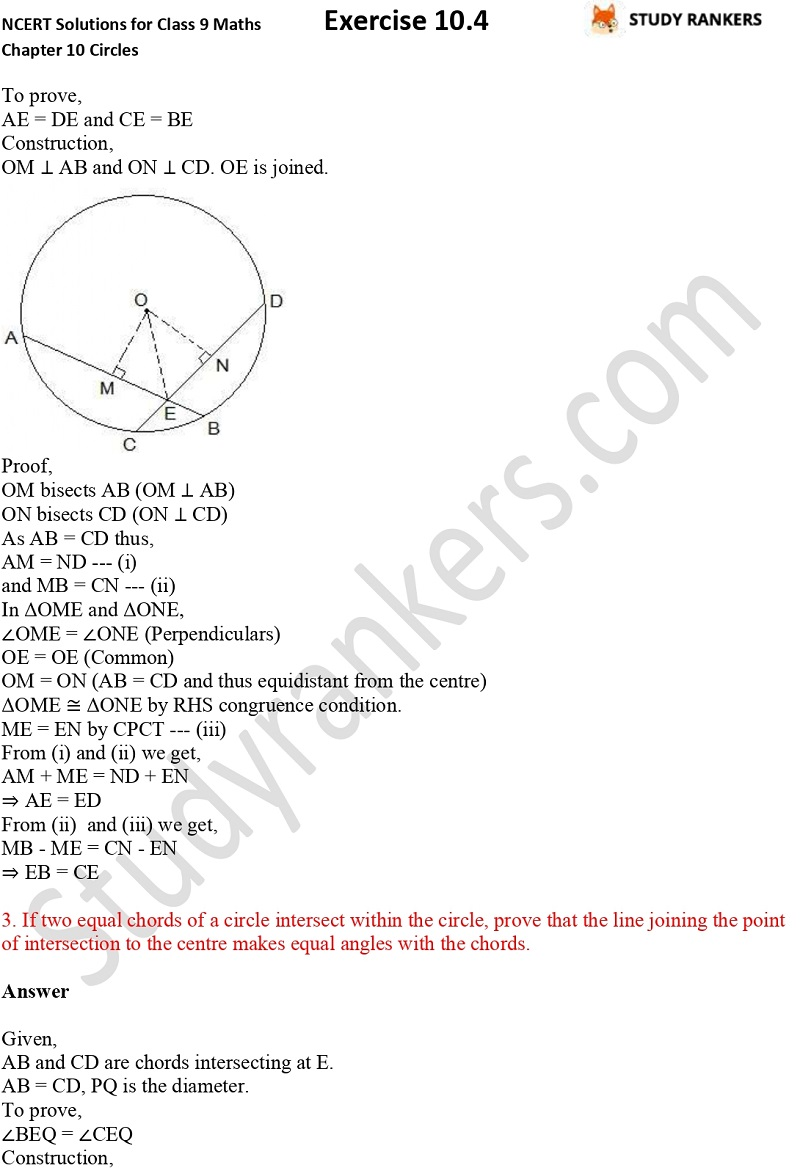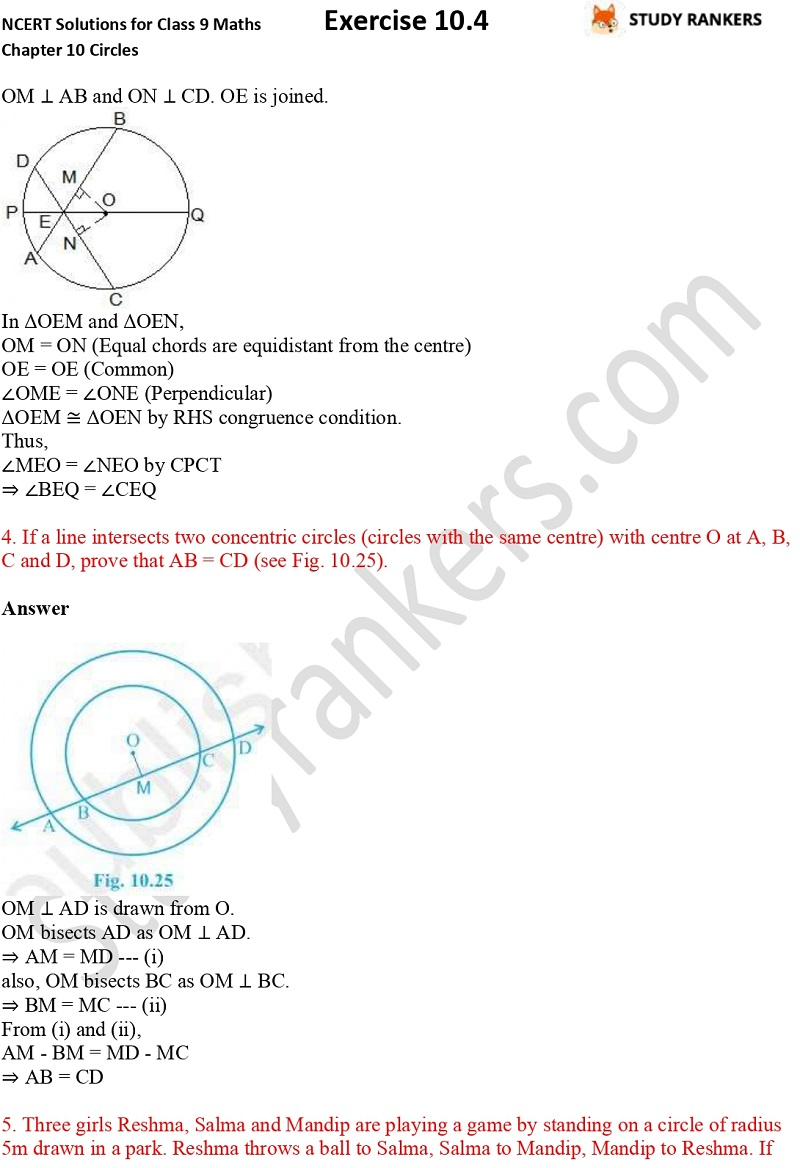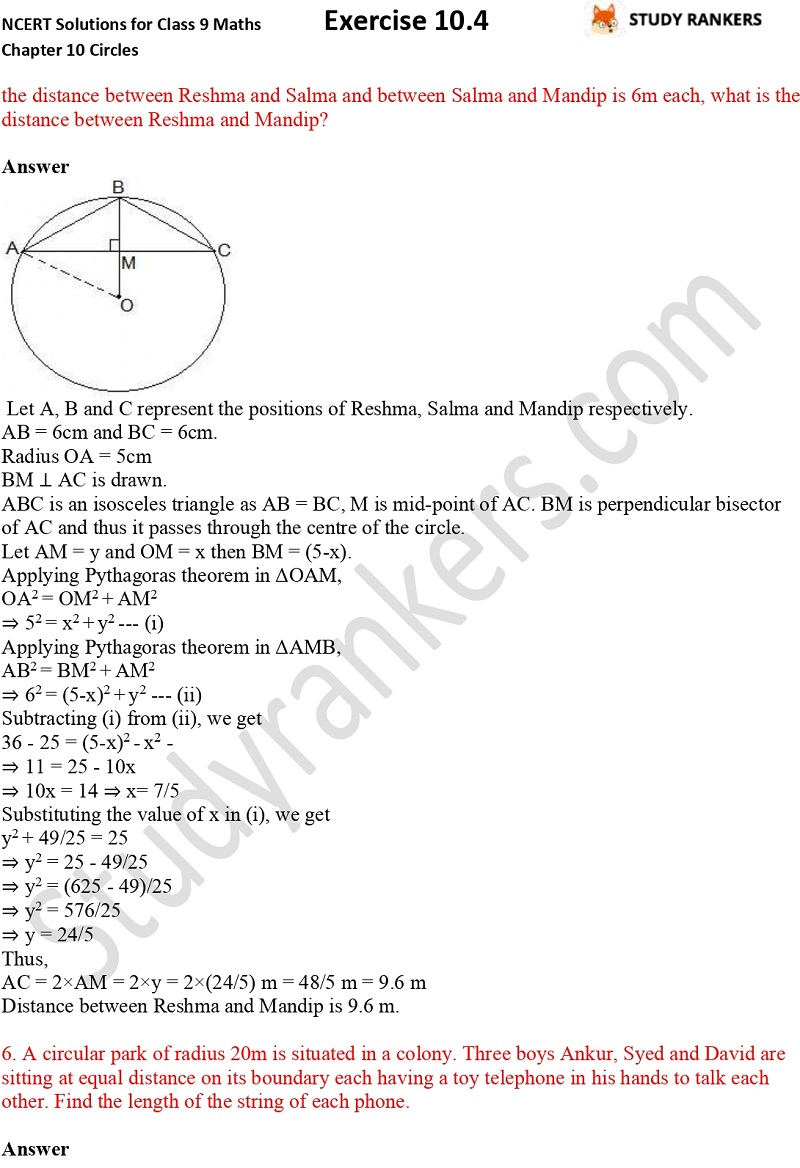>

## NCERT Solutions for Class 9 Maths Chapter 10 Circles Exercise 10.4

If you are looking for Chapter 10 Circles Exercise 10.4 NCERT Solutions for Class 9 Maths then your search ends here as you will find accurate and detailed answers of every questions of Ex 10.4 on this page. These Class 9 Maths NCERT Solutions are prepared by Studyrankers subject matter experts which will help you in understanding the application of formulas and boost your morale. Important points and formulas provided in this chapter will be useful for you in higher classes so you must build your basic fundamentals.

There are six questions in Exercise 10.4 which are about finding the length of the common chord, proving the segments of one chord are equal to corresponding segments of the other chord.X